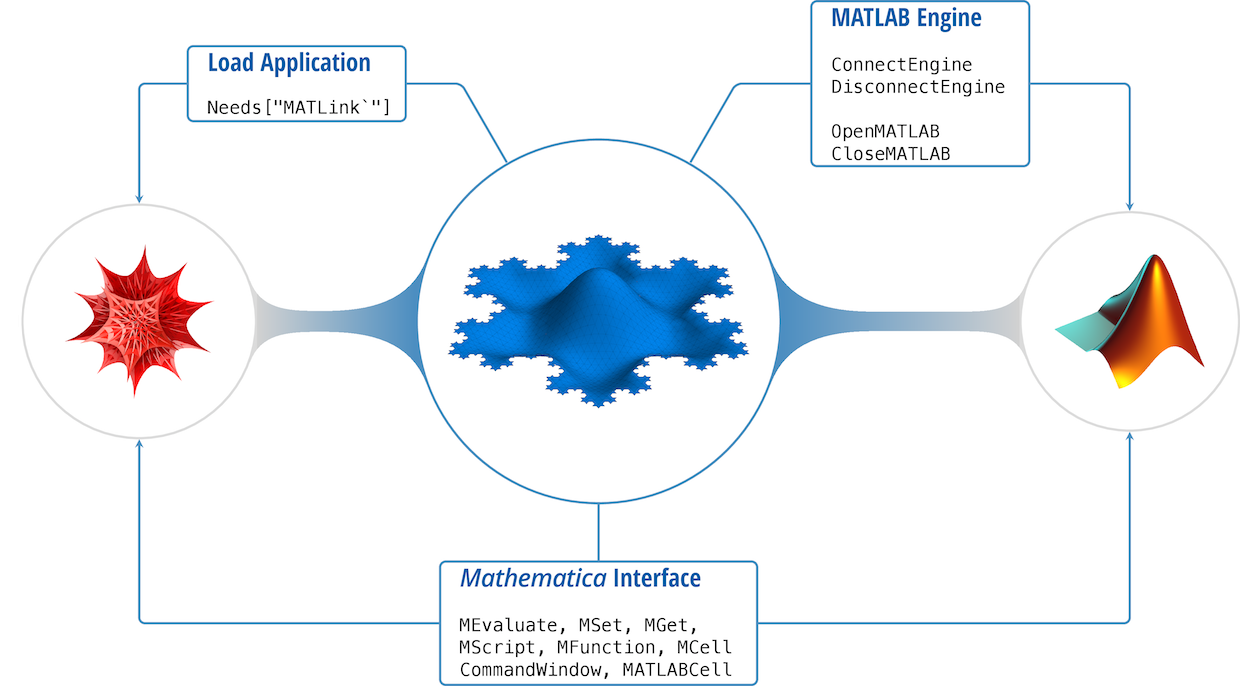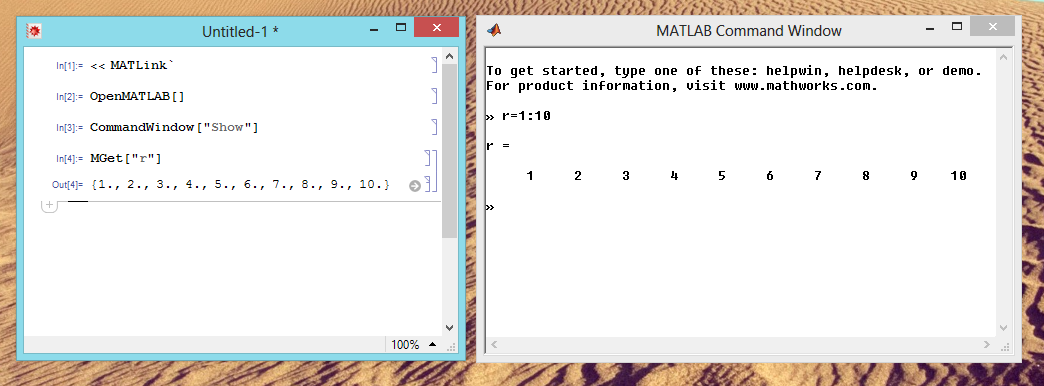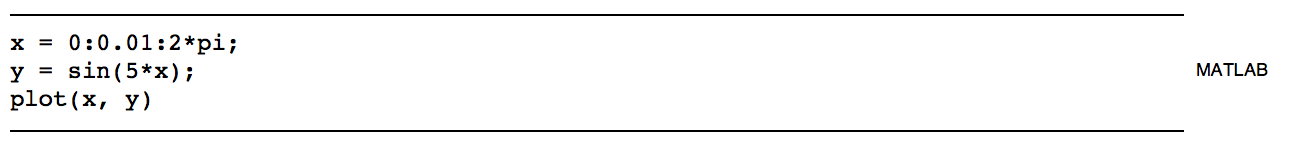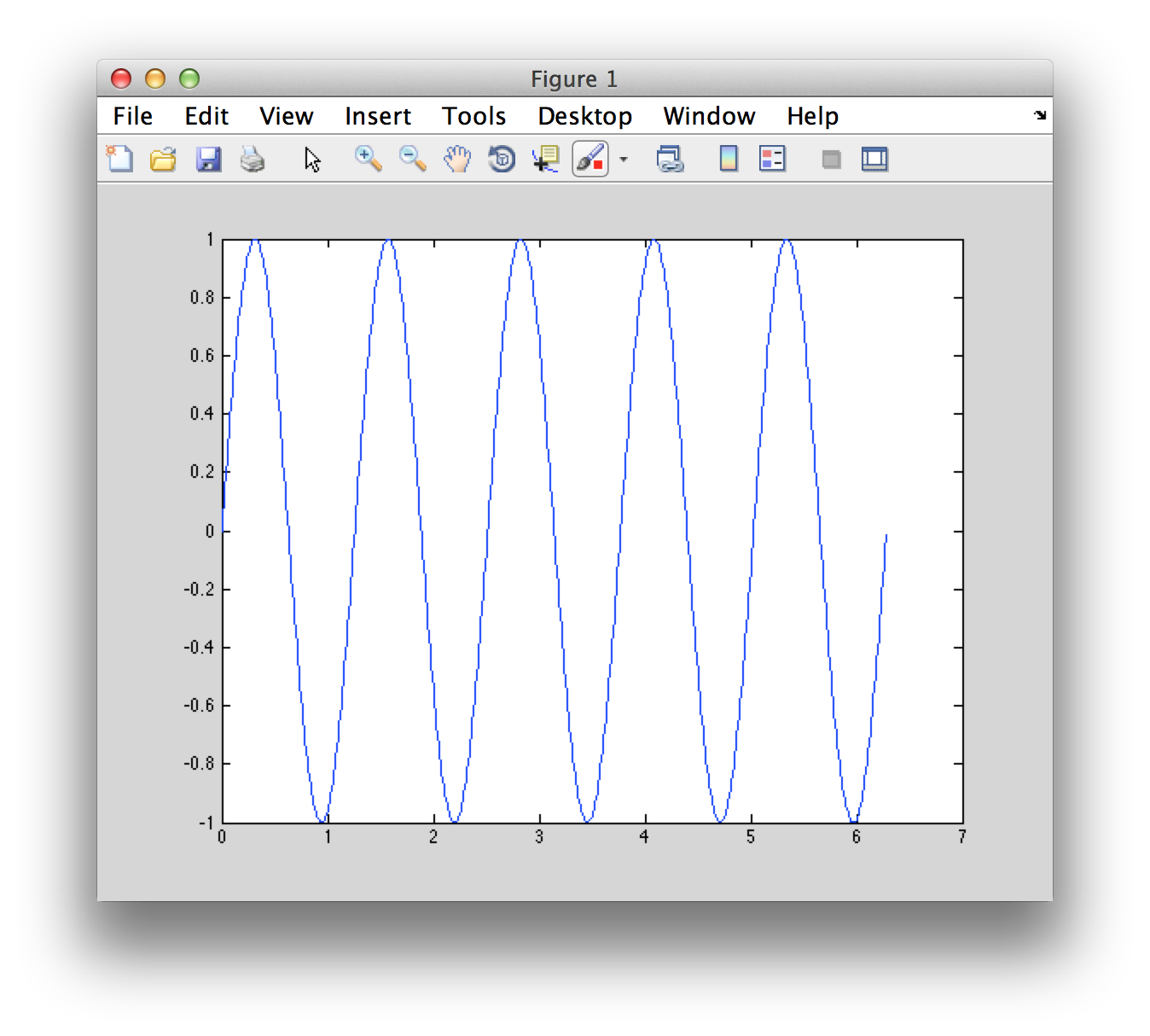# Documentation

MATLink is a Mathematica application to communicate with MATLAB, providing functionality to easily transfer data between the two systems, and use MATLAB functions, scripts and toolboxes from within Mathematica. MATLink is not a substitute for MATLAB, nor is it a replacement for actual knowledge of MATLAB and Mathematica; it is merely an application that allows the user to harness the power of both computational systems to program and develop more efficiently.

MATLink is written in Mathematica and C++ and connects to MATLAB using the MATLAB Engine interface. The image to the right is an overview of the different functions in MATLink and how they interact with the different systems. This documentation contains## Function Reference

### Connecting to MATLAB

MATLink has four functions that handle various aspects of startup and shutdown of MATLAB and the underlying engine

OpenMATLAB, automatically calls ConnectEngine if the engine is not running, so one can launch MATLAB directly with it after loading the package. Similarly, DisconnectEngine will automatically terminate any open connection to MATLAB before shutting down the engine.

MATLink uses a session folder in $TemporaryDirectory to save scripts and functions defined during the current session. These are removed when DisconnectEngine is called, or by the operating system (typically after a reboot or daily, depending on the system), if there are any abandoned directories after a crash. To manually remove those folders, run MATLinkDeveloperCleanupTemporaryDirectories[]. ConnectEngine[] will connect to the MATLAB engine using MathLink. #### Details & Options • On linux and OS X, if the mengine binary is not found, ConnectEngine will try to auto compile it. • ConnectEngine creates a session directory in $TemporaryDirectory to which MScripts are written. This directory is automatically removed when DisconnectEngine is called.
DisconnectEngine[] will terminate the MathLink connection with the MATLAB engine.

#### Details & Options

• If a MATLAB connection is alive, DisconnectEngine will terminate it before shutting down the engine.
• DisconnectEngine deletes the session directory in $TemporaryDirectory that was created by ConnectEngine. OpenMATLAB[] will start an instance of MATLAB for use with MATLink. #### Details & Options • If the MATLAB engine is not running, OpenMATLAB will call ConnectEngine[] to launch the engine before starting MATLAB CloseMATLAB[] will close the currently open instance of MATLAB. ### Evaluating MATLAB commands Arbitrary MATLAB code can be evaluated in three ways — using MEvaluate, MATLABCell or CommandWindow (only the first two are available on non-Windows operating systems). The primary difference between the three functions is: • MEvaluate is a Mathematica function that takes MATLAB input as strings, and can be conveniently used in larger programs • MATLABCell is a styled cell in a Mathematica notebook, that is useful for interactive work • CommandWindow is just a Mathematica function that activates a native MATLAB command window interface. With this, one works directly with MATLAB, independent of MATLink (although the variables are still accessible via MATLink). Since there is overhead in transferring instructions and output with MEvaluate, it is advisable to not display output where not necessary (both, in MATLAB and Mathematica). If you need to run the same set of instructions repeatedly, use an MScript instead of MEvaluate. MEvaluate["code"] will execute code in MATLAB as if it were entered at the command prompt and returns the output as text. #### Details & Options • MEvaluate, when successful, will always return a string containing the output from MATLAB's command window. #### Possible issues • MEvaluate performance suffers if the output is not suppressed in MATLAB code. If you do not need to see the output of MEvaluate, use MEvaluate["command;"] instead of MEvaluate["command"];. • The output length of MEvaluate is limited to approximatey 100,000 characters. The rest will be truncated. CommandWindow["action"] will perform "action" on MATLAB's built-in command window. This function works only on Windows.#### Details & Options • CommandWindow["Show"] displays the command window. When an evaluation is not in progress, this window can be used to input MATLAB commands independently of MATLink. • CommandWindow["Hide"] hides the command window. MATLABCell[] creates a program cell in the current notebook, whose contents are interpreted as MATLAB code.### Transferring variables Any supported datatype can be transferred from Mathematica to MATLAB and vice versa using MSet and MGet respectively. However, one must be aware of the overhead involved in data transfer (when using any link technology) and avoid programming pitfalls that result in unnecessary back-and-forth data transfer (unless absolutely necessary). It is always good practice to transfer as much as possible in one go, and only transfer what is necessary. Every effort has been made to convert quantities to native data structures in each system (such as arrays in MATLAB and lists in Mathematica) and allow the user to retrun the same data to the originating system without loss of structure, but there are certain exceptions (MATLAB cells). See the translation rules for more information. MGet["var"] will return the value of the variable var from the MATLAB workspace. Data structures are translated into a Mathematica compatible format. #### Details & Options • var must be a string denoting the name of the variable in MATLAB • MEvaluate["x = 1:10;"] MGet["x"] (* Out= {1., 2., 3., 4., 5., 6., 7., 8., 9., 10.} *)  • MGet is Listable • MEvaluate["[v, e] = eig(rand(5));"] {v, e} = MGet[{"v", "e"}]  #### Possible issues • MATLAB works with floating point values by default. Even array indices are floating point, and such values need to be explicitly rounded in Mathematica before they can be used as indices again. MEvaluate["s = size(zeros(3,4));"] s = MGet["s"] (* Out: {3., 4.} *)  • Do not attempt to use MGet on objects (classdef objects) or data structures which contain objects. This will crash MATLAB because of a MATLAB bug. See the known issues section for additional details. MSet["var", value] will assign value to variable var in the MATLAB workspace. #### Examples • MSet["a", {1,2,3}] MEvaluate["a"] (* Out: a = 1 2 3 *)  #### Details & Options • var must be a string denoting the name of the variable in MATLAB • value must be in a MATLink compatible format. • To force a list to be interpreted as a MATLAB cell, wrap it in the MCell head: • MSet["a", MCell[{1, 2, 3}]] MEvaluate["a"] (* Out: a =    *)  MCell is an inert head used with MSet to indicate that the list be interpreted as a MATLAB cell data structure. #### Example MSet["a", MCell[{1, 2, 3}]] MEvaluate["a"] (* Out: a =    *)  ### Scripts & Functions MATLAB programming involves script files, which are a series of sequential instructions to the interpreter (executed in the base workspace) or functions, which take zero or more inputs and return zero or more outputs (executed in its own workspace). The MScript and MFunction functions allow one to execute MATLAB scripts and functions from within Mathematica. This makes it easy to reuse code found online, either on the MathWorks File Exchange or elsewhere, instead of reimplementing it in Mathematica. In MATLAB, one can define functions to have completely different behaviour based on the number of output arguments for the same set of input arguments. This is at odds with the behaviour in Mathematica (and in functional programming languages in general), where a function's behaviour is determined solely by its inputs. To bridge this divide, MFunction offers the functionality to use the multiple output form of MATLAB functions, but the number of outputs must be set explicitly when defining the function. MScript["scriptname", "commands"] will create a MATLAB script containing commands. #### Details & Options • MScript["scriptname", "commands"] returns MScript["scriptname"], which can be evaluated using MEvaluate. • hello = MScript["hello", "disp('Hello world!')"]; MEvaluate@hello (* Out = "Hello world!" *)  • When creating new scripts, MScript will not overwrite exsiting .m files. To force overwrite an existing file, use the option "Overwrite" -> True (default value for "Overwrite" is False). MFunction["func"] represents a MATLAB function func (that is on MATLAB's path), and can be called directly from within Mathematica. MFunction["func", "body"] creates a new .m file with the contents body and returns MFunction["func"]. #### Examples • Calling a built-in MATLAB function eig = MFunction["eig"]; eig[{{1, 2}, {3, 4}}] (* Out= {{-0.372281}, {5.37228}} *)  • Creating a custom function and using it add = MFunction["add", " function res = add(x,y) res = x+y end "]; add[3, 4] (* Out = 7 *)  • Creating an anonymous function and using it add = MFunction["add", "@(x,y)x+y"]; add[3, 4] (* Out = 7 *)  Anonymous functions defined in this manner form a closure, as in MATLAB. #### Details & Options • Use MFunction["func", "OutputArguments" -> n] when func returns more than one argument (default value for "OutputArguments" is 1). • eigsys = MFunction["eig", "OutputArguments" -> 2]; eigsys[{{1, 2}, {3, 4}}] (* Out= {{{-0.824565, -0.415974}, {0.565767, -0.909377}}, {{-0.372281, 0.}, {0., 5.37228}}} *)  • If func returns no outputs (or to suppress output), use MFunction["func", "Output" -> False] (default value for "Output" is True). • imagesc = MFunction["imagesc", "Output" -> False]; data = Import["ExampleData/ozonemap.hdf", {"Datasets", "TOTAL_OZONE"}][[20 ;;, All]]; imagesc[data];  Note that MATLAB plots will be opened in a new window, unlike Mathematica plots which are displayed inside the notebook. • When creating new functions using the syntax MFunction["func", "body"], MFunction will not overwrite exsiting .m files. To force overwrite an existing file, use the option "Overwrite" -> True (default value for "Overwrite" is False). • If the first character in "body" is @, then the function is defined as an anonymous function in MATLAB's workspace and no function file is created. #### Possible issues • By default MFunction assumes a single output argument, which causes errors in some MATLAB functions that return no output. • MFunction["disp"]["Hello"] MATLink::errx: Error using disp Too many output arguments. (* Out=$Failed *)

Use "Output" -> False in such situations.

## Supported Datatypes

The following data types can be transferred in both directions:

• double precision numerical arrays (including multidimensional)
• double precision sparse matrices
• logical arrays (including multidimensional)
• sparse logical matrices
• strings (i.e. char arrays of dimension [1 n])
• cells (including multidimensional)
• structs (only with size [1 1])

The following can only be transferred from MATLAB to Mathematica:

• numerical arrays with the following types: single, int8, int16, int32, uint8 and uint16
• structs with any number of elements

### Rules for translating data structures

This section documents the internal rules that govern the translation of data structures from MATLAB to Mathematica and vice versa. You do not need to do this conversion, but you should be aware of how it is converted so that you can design your programs accordingly.

#### MATLAB → Mathematica

1. ##### Numerical Arrays
• single and double are converted to Real or Complex (as the case may be)
• All the supported int* and uint* are converted to Integer or Complex (as the case may be)
• 1×1 matrices (scalars) are atomic
• 1×N matrices (row vectors) are simple (packed) lists of length N
• M×N×... matrices (any dimension) are packed arrays of the same dimension
• Empty matrices (any dimension) are {}
2. ##### Logical Arrays
• 0 and 1 (logical) or true and false are converted to True and False respectively
• All other rules are identical to those for numerical arrays
3. ##### Char Arrays
• char is converted to String
• 1×N char arrays are atomic strings
• M×N×... char arrays (any dimension) are lists of individual characters
4. ##### Struct Arrays
• struct is converted to a list of rules
• Field names are the LHS of rules (stored as string); values are the RHS of rules
• 1×1 struct arrays are a simple list of rules of length F, where F is the number of fields
• 1×N struct arrays are a list of rules of dimension N×F
• M×N×... struct arrays (any dimension) are lists of dimension M×N×...×F
5. ##### Cells
• 1×1 cells are atomic
• 1×N cells are simple (unpacked) lists of length N
• M×N×... cells (any dimension) are unpacked arrays
• Empty cells (any dimension) are {}

#### Mathematica → MATLAB

1. ##### Numbers and numerical lists
• Integer, Real and Complex are all converted to double
• Simple lists are row vectors
• All other rectangular lists of lists (any dimension) are converted to an identical sized array.
• Numerical lists can be interpreted as MATLAB cells using the MCell head
2. ##### Strings and string lists
• String is converted to a 1×N char array, where N is the string length.
• Lists of strings (any dimension) are converted to cell arrays of appropriate dimensions
3. ##### Lists of rules
• Simple lists of rules are converted to a struct if all the LHS of the rules is a string, the RHS is a supported datatype and there are no duplicate fields.
4. ##### Mixed lists
• Lists of mixed datatypes are transferred as cells of appropriate dimensions

## Known Issues

• #### MATLAB R2014a

The OS X (and possibly Linux) versions of MATLAB R2014a contain a bug that breaks MATLink. Unfortunately this bug is in a core MATLAB Engine feature MATLink relies on, and it is not easy to work around it. Until this is resolved, please consider using earlier versions of MATLAB (R2013b or earlier) on OS X or Linux to run MATLink.

#### Large array support

At the moment, only arrays with less than 2^31-1 elements are supported. Note that this is true for matrices and multidimensional arrays as well: the total number of matrix elements may not excede 2^31-1 even if the matrix has fewer rows and columns than this. As an example, the largest supported square matrix can be of size 46341 by 46341.

As a reference point, a double precision array with the maximum number of allowed elements would take up 16 GB of memory, so this limit should be more than sufficient for most applications.

• #### Inf and NaN

Inf and Nan are not supported at the moment. The values returned to Mathematica are not safe to use: operations on them give unpredictable results.

• #### Multiple instances of MATLAB

If a MATLAB background process has already been started by MATLink, it will not be possible to launch another instance of MATLAB by clicking on its icon. As a workaround, either start MATLAB before you call OpenMATLAB[] or launch the MATLAB executable directly as:

##### On OS X
open -n /Applications/MATLAB_R2013a.app


or by executing the binary from the command line

/Applications/MATLAB_R2013a.app/bin/matlab

##### On Windows

Run the binary located at

C:\Program Files\MATLAB\R2013a\bin\matlab.exe

• #### Transferring MATLAB objects

Do not use MGet on MATLAB objects, or data structures that contain custom classes as elements. On OS X and Unix this will crash the MATLAB process because of a bug in the MATLAB Engine interface. For example,

m = containers.Map('a',1);
s = struct('a',1, 'b',m);


Now running MGet["m"] will crash MATLAB because m is an object. MGet["s"] will crash because s contains an object.

• #### Reading HDF5 based .mat files

All the limitations of the MATLAB Engine interface apply to MATLink. The most noticeable of these is that HFD5 based .mat files cannot be read. Quoting the MATLAB documentation,

The MATLAB engine cannot read MAT-files in a format based on HDF5. These are MAT-files saved using the -v7.3 option of the save function or opened using the w7.3 mode argument to the C or Fortran matOpen function.

As of R2013a, MATLAB does not save .mat files in this format by default, unless its settings are changed.

• #### Unicode support

MGet and MSet do support Unicode strings, and will preserve Unicode characters. However, MEvaluate will not preserve unicode characters in its output. MEvaluate should handle Unicode input correctly. If you discover a situation where it does not, please report it.

The reason unicode output needed to be disabled for MEvaluate is that MATLAB's C API is unpredictable and may not produce correct unicode output depending on version and operating system.

MEvaluate["s='Paul Erdős'"] (* Unicode input *)
(* Out: s = Paul Erd!s *)

MGet["s"]
(* Out: Paul Erdős *)


In MEvaluate's output Unicode in mangled, however, MGet transfers it correctly.

A workaround is using evalc. This is not used in MATLink because of unsolved issue #29.

• #### Path names with non-ASCII characters on Windows

On Windows, it maybe not be possible to change (cd) MATLAB into directories that have non-ASCII characters in their path name. At the moment, the following workaround is available:
MEvaluate["feature('DefaultCharacterSet', 'windows-1252')"]
MFunction["cd"]["\\path\\to\\directory"]

It may be necessary to use your system's default encoding instead of windows-1252. To find what it is, evaluate feature('DefaultCharacterSet') in a standalone (non-MATLink) MATLAB session.